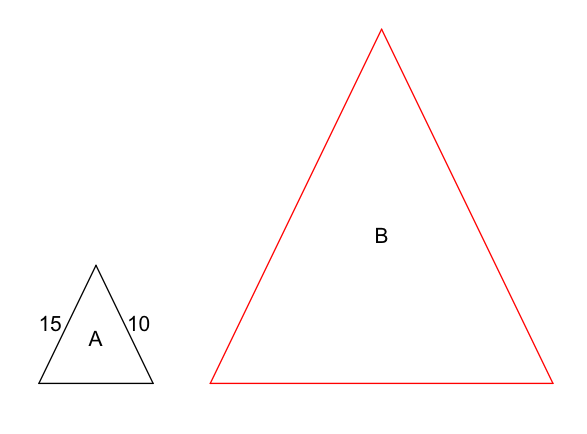## Example Questions

### Example Question #1 : How To Find If Two Acute / Obtuse Triangles Are Similar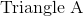and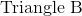are similar triangles.  The perimeter of Triangle A is 45” and the length of two of its sides are 15” and 10”.  If the perimeter of Triangle B is 135” and what are lengths of two of its sides?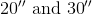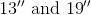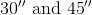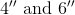Explanation:

The perimeter is equal to the sum of the three sides.  In similar triangles, each side is in proportion to its correlating side.  The perimeters are also in equal proportion.

Perimeter A = 45” and perimeter B = 135”

The proportion of Perimeter A to Perimeter B is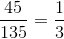This applies to the sides of the triangle.  Therefore to get the any side of Triangle B, just multiply the correlating side by 3.

15” x 3 = 45”

10” x 3 = 30“